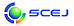English page## \$B4pD49V1i!&>7BT9V1i!&E8K>9V1i!&0MMj9V1i!&;:6H%;%C%7%g%s(B:\$BAm3g9V1i(B

\$B:G=*99?7F|;~!'(B2015-03-05 09:42:37

### \$BBh(B 1 \$BF|(B ( 3/19 \$BLZ(B )

\$B4pD49V1i(B
Z \$B2q>l(B
Z106 \$B!V\$\$\$^2=3X;:6H\$O2?\$rL\;X\$9\$+!W(B (11:00\$B!A(B12:00)

(\$BF|K\2=3X9)6H6(2q(B) \$B@>=P(B \$BE0M:(B \$B;a(B
\$B>7BT9V1i(B
E \$B2q>l(B
E121 \$B!V%^%\$%/%mGH%(%M%k%.!<\$N2=3X%W%m%;%9\$X\$NE,MQ3+H/!W(B (15:20\$B!A(B15:50)

(\$B%"%j%*%9(B) \$B:4F#(B \$B?J(B \$B;a(B
E122 \$B!V?77?F0J*:YK&G]M\MQ%P%\$%*%j%"%/%?!<\$N6&F13+H/!W(B (15:50\$B!A(B16:20)

(\$BF|4x(B) \$BED86(B \$BD>
E123 \$B!V;:3XO"7H\$K\$h\$k%P%\$%*G3NA@=B\$%7%9%F%`\$N3+H/;vNc!W(B (16:20\$B!A(B16:50)

(Bio-energy) \$Bzp(B \$B??;J(B \$B;a(B
F \$B2q>l(B
F113 \$B!V%R%e!<%^%s%(%i!<\$H0BA4!W(B (13:00\$B!A(B13:40)

(\$B;:Am8&(B) \$BCfED(B \$B5|(B \$B;a(B
F116 \$B!V1?E>71N}%7%_%e%l!<%?!<\$N5;=QF08~!W(B (14:00\$B!A(B14:20)

(\$B%7%e%J%\$%@!<%(%l%/%H%j%C%/(B) \$BNS(B \$B=E8w(B \$B;a(B
F117 \$B!V%3%s%T%e!<%?%0%i%U%#%C%/%9(B(CG)\$B\$N3hMQ\$K\$h\$kBN46650i!W(B (14:20\$B!A(B14:40)

(\$BF|4x(B) \$B@>;3(B \$BJ8M:(B \$B;a(B
G \$B2q>l(B
G113 \$B!VEl3\$F;?744@~\$HD6EEF3%j%K%"\$N5;=Q3+H/!W(B (13:00\$B!A(B13:40)

(\$BEl3\$N95RE4F;(B) \$BB-N)(B \$B>;?N(B \$B;a(B
G117 \$B!V!H(BQMONOS\$B!I(B \$B (14:20\$B!A(B15:00)

(\$B%9%Q%\$%P!<(B) \$B4X;3(B \$BOB=((B \$B;a(B
G119 \$B!V2=3X;:6H\$N>-Mh (15:00\$B!A(B15:40)

(\$B?72=3X5;=Q?d?J6(2q(B) \$B8^==Mr(B \$BL@(B \$B;a(B
G121 \$B!V?M9)8w7??"J*9)>l\$N8=>u\$H2]Bj(B \$B!W(B (15:40\$B!A(B16:20)

(\$B?"J*9)>l8&5f2q(B) \$B8E:_(B \$BK-
H \$B2q>l(B
H119 \$B!V%7%'!<%k3WL?\$HF|K\4k6H\$N%S%8%M%9%A%c%s%9!W(B (15:00\$B!A(B15:40)

(\$BEl%l7P1D8&5f=j(B) \$BJ!ED(B \$B2BG7(B \$B;a(B
\$BE8K>9V1i(B
H \$B2q>l(B
H113 \$B!V%7%'!<%k%,%9\$N8=>u\$HE8K>!W(B (13:00\$B!A(B14:20)

(\$B;00fJ*;:(B) \$B0\$It(B \$BF;M:(B \$B;a(B
\$B0MMj9V1i(B
E \$B2q>l(B
E113 \$B!V%5%?%1\$K\$*\$1\$k (13:00\$B!A(B13:30)

(\$B:4C]2=3X5!3#9)6H(B) \$B!{:,K\(B \$B9'9((B \$B;a(B\$B!&(B \$B2CF#(B \$B9%0l(B \$B;a(B
E114 \$B!V>=@OA`:n\$K\$*\$1\$k3IYB!&:.9g\$NLr3d!W(B (13:30\$B!A(B14:00)

(\$B7nEg5!3#(B) \$B?\ED(B \$B1Q4u(B \$B;a(B
E115 \$B!V3IYBAe7?H?1~5!\$N3IYB5;=Q(B(\$B%]%j%^!<=E9g5!\$rCf?4\$K(B)\$B!W(B (14:00\$B!A(B14:30)

(\$B?@9]4D6-%=%j%e!<%7%g%s(B) \$B!{Fn(B \$B=S=<(B \$B;a(B\$B!&(B \$B;3It(B \$BK'(B \$B;a(B\$B!&(B \$B5FCS(B \$B2mI'(B \$B;a(B
G \$B2q>l(B
G115 \$B!V\$3\$l\$+\$i\$N2=3XIJ86NA8;\$NA*Br(B-\$B2=3X;:6H\$,?MN`\$K9W8%\$7B3\$1\$k\$?\$a\$KI,MW\$J5;=Q!W(B (13:40\$B!A(B14:20)

(\$B;00f2=3X(B) \$B!{F#ED(B \$B>HE5(B \$B;a(B\$B!&(B \$B;T@n(B \$B??0lO:(B \$B;a(B
G123 \$B!V%+%M%+\$N(BR\$B!u(BD\$B@oN,\$H\$=\$NE8K>(B \$B!AEE;R:`NAJ,Ln\$N3+H/;vNc\$rCf?4\$K!A!W(B (16:20\$B!A(B17:00)

(\$B%+%M%+(B) \$B1JLn(B \$B9-:n(B \$B;a(B
\$B;:6H%;%C%7%g%s(B:\$BAm3g9V1i(B
Z \$B2q>l(B
Z125 \$B!VF|;:<+F0 (17:00\$B!A(B18:00)

(\$BF|;:<+F0

### \$BBh(B 2 \$BF|(B ( 3/20 \$B6b(B )

\$B>7BT9V1i(B
F \$B2q>l(B
F205 \$B!V%F%i%X%k%DGH\$rMQ\$\$\$?HsGK2u;n83!W(B (10:20\$B!A(B10:40)

(JFE\$B%F%/%N%j%5!<%A(B) \$B;38}(B \$BATFsO/(B \$B;a(B\$B!&(B \$B!{8WHx(B \$B>4(B \$B;a(B
F206 \$B!V@V30@~%+%a%i\$K\$h\$k7WB,5;=Q!W(B (10:40\$B!A(B11:00)

(JFE\$B%F%/%N%j%5!<%A(B) \$BFsB<(B \$BCR><(B \$B;a(B
F216 \$B!V(BEncapsulation of enzyme by spray drying: Different formulation of feed liquid\$B!W(B (14:00\$B!A(B14:20)

(Chula U.) \$B!{(BSoottitantawat Apinan \$B;a(B\$B!&(B (NSTDA, Thailand) Ruktanonchai Uracha \$B;a(B\$B!&(B (Kagawa U.) Yoshii Hidefumi \$B;a(B
F218 \$B!V(BDevelopment of inulin powder production process from Jerusalem artichoke (Helianthus tuberosus L.) tubers and quality determination\$B!W(B (14:40\$B!A(B15:00)

(Kasetsart U.) \$B!{(BKhuenpet K. \$B;a(B\$B!&(B Jittanit W. \$B;a(B\$B!&(B Sirisansaneeyakul S. \$B;a(B\$B!&(B (TUMSAT) Fukuoka M. \$B;a(B
F220 \$B!V(BProduction of carbohydrate products from tropical fruit wastes by subcritical water treatment\$B!W(B (15:20\$B!A(B15:40)

(Silpakorn U.) \$B!{(BKhuwijitjaru Pramote \$B;a(B\$B!&(B Klinchongkon Khwanjai \$B;a(B\$B!&(B Pokpong Areewan \$B;a(B\$B!&(B (Kyoto U.) Adachi Shuji \$B;a(B
F223 \$B!V(BComputer simulation in thermal food processing\$B!W(B (16:20\$B!A(B17:20)

(\$B3\$MNBg(B) \$B:(B \$B;a(B
G \$B2q>l(B
G204 \$B!V(BThermochemical conversion of woody biomass into chemicals\$B!W(B (10:10\$B!A(B10:30)

(Kyoto U.) Hasegawa Isao \$B;a(B
G206 \$B!V(BAn Integrated-Thinking Design for Biological C, N, S Removal from TFT-LCD Wastewaters -Will The Dream Come True?\$B!W(B (10:30\$B!A(B11:10)

(Nat. Cheng Kung U.) Whang Liang-Ming \$B;a(B
G208 \$B!V(BPrecious metal recovery using modified biomass waste\$B!W(B (11:10\$B!A(B11:30)

(Saga U.) Kawakita Hidetaka \$B;a(B
G209 \$B!V(BDevelopment of biomass residue recycle system of plantation based on the analyses of material flow and soil ecosystem\$B!W(B (11:30\$B!A(B11:50)

(Yokohama Nat. U.) Fujie Koichi \$B;a(B
G214 \$B!VJ!Eg4D6-=\$I|\$HGQ;_A (13:20\$B!A(B14:00)

(\$BEENOCf1{8&5f=j(B) \$B!{0f>e(B \$B@5(B \$B;a(B\$B!&(B (\$B%(%M%k%.!
G216 \$B!VJ| (14:00\$B!A(B14:40)

(\$BD92,5;2JBg(B) \$B4];3(B \$B5W0l(B \$B;a(B
G218 \$B!V1x@w?eBP:v!&G3NA%G%V%j (14:40\$B!A(B15:20)

(\$BAaBg(B/\$BCOHW9)3X2q(B) \$B>.Jw(B \$B=(M:(B \$B;a(B
G220 \$B!VJ|m!"GQ4~J*\$NE,@5=hM}5;=Q!W(B (15:20\$B!A(B16:00)

(\$B9qN)4D6-8&(B) \$BBgGw(B \$B@/9@(B \$B;a(B
H \$B2q>l(B
H214 \$B!V(BComplex hydrides as solid-state electrolytes for rechargeable batteries\$B!W(B (13:20\$B!A(B14:00)

(Tohoku U.) Unemoto Atsushi \$B;a(B
H216 \$B!V(BOrdered mesoporous carbon-supported nano-platinum: Promising anodic catalyst for direct methanol fuel\$B!W(B (14:00\$B!A(B14:40)

H219 \$B!V(BThe rational design of materials for intensified reaction and separation in energy-related processes\$B!W(B (15:00\$B!A(B15:40)

(Newcastle U.) Metcalfe Ian \$B;a(B
H221 \$B!V(BWater/steam electrolysis for hydrogen production\$B!W(B (15:40\$B!A(B16:20)

(Kyushu U.) Matsumoto Hiroshige \$B;a(B
N \$B2q>l(B
N202 \$B!V=w@-\$N3hLvB%?J\$H%\$%N%Y!<%7%g%s\$K\$h\$k9,J! (9:20\$B!A(B10:00)

(JAXA) \$B1vK~(B \$BE5;R(B \$B;a(B
N204 \$B!VD4M}2J3X\$H\$H\$b\$K!W(B (10:00\$B!A(B10:40)

\$B:4F#(B \$B=(H~(B \$B;a(B
N206 \$B!V%0%m!<%P%k2=;~Be\$N=w@-\$N%(%s%Q%o!<%a%s%H!W(B (10:40\$B!A(B11:20)

(\$B
\$BE8K>9V1i(B
F \$B2q>l(B
F213 \$B!V(BOverview of Recent Developments in Drying Technology\$B!W(B (13:00\$B!A(B13:40)

(King Mongkut's U.) Sakamon Devahastin \$B;a(B
\$B0MMj9V1i(B
F \$B2q>l(B
F201 \$B!V2=3X9)>l\$G;HMQ\$5\$l\$?(BFRP\$B5!4o\$NNt2=!&B;=};vNc!W(B (9:00\$B!A(B9:20)

(\$B=;M'2=3X(B) \$BCfED(B \$B44=S(B \$B;a(B
F202 \$B!VBQ?)(BFRP\$B5!4o\$N5,3J4p=`\$HNt2=?GCG(B-\$BBQ?)CyAe(BJIS\$B2~@5\$H@=:n\$NMWE@!W(B (9:20\$B!A(B9:40)

(\$B6/2=%W%i%9%A%C%/6(2qBQ?)It2q(B) \$BCf0f(B \$BK.I'(B \$B;a(B
F203 \$B!V(BFRP\$B5!4o\$ND62;GH\$K\$h\$kNt2=?GCG!W(B (9:40\$B!A(B10:00)

(\$BEl9)Bg1!(B) \$B!{5WJ]Fb(B \$B>;IR(B \$B;a(B\$B!&(B (\$BF|Bg@8;:9)(B) \$B
F204 \$B!V(BFRP\$BJd6/1v%S4I\$ND62;GH8|\$5B,Dj!W(B (10:00\$B!A(B10:20)

(\$B>
F207 \$B!V\$U\$CAG (11:00\$B!A(B11:20)

(\$B%K%A%"%9(B) \$B>.NS(B \$B1Q
N \$B2q>l(B
N212 \$B!VJ,;RG'<1@-9bJ,;RB?9&:`NA\$K\$h\$kJ,N%:`NA\$N3+H/!W(B (13:00\$B!A(B13:20)

(\$B6eBg(B) \$B;01:(B \$B2B;R(B \$B;a(B
N213 \$B!V9ZAGH?1~@-\$rM-\$9\$kN>?FG^@-%Z%W%A%I:`NA\$N3+H/!W(B (13:20\$B!A(B13:40)

(\$B6eBg(B) \$B
N214 \$B!V%Z%W%A%I%"%l%\$\$rMQ\$\$\$?%(%T%H!<%W2r@O\$K\$h\$k?)J*%"%l%k%.!<\$N7P2a!&<#NEM=B,!W(B (13:40\$B!A(B14:00)

(\$BEl9)Bg(B) \$BBg2OFb(B \$BH~F`(B \$B;a(B
N215 \$B!V%j%]%=!<%`\$rMQ\$\$\$?I{:nMQ\$N\$J\$\$\$,\$s<#NE\$rL\;X\$7\$?E83+!W(B (14:00\$B!A(B14:20)

(\$B?r>kBg1~MQ@8L?(B) \$B>>K\(B \$BM[;R(B \$B;a(B
N216 \$B!V:YK&%^%\$%/%m%"%l%\$\$rMxMQ\$7\$?7lCf=[4D (14:20\$B!A(B14:40)

(\$BG@9)Bg(B) \$B5HLn(B \$BCN;R(B \$B;a(B

### \$BBh(B 3 \$BF|(B ( 3/21 \$BEZ(B )

\$B>7BT9V1i(B
I \$B2q>l(B
I301 \$B!V (9:00\$B!A(B9:40)

(\$BAaBg(B) \$B1|B<(B \$BD>;N(B \$B;a(B
I303 \$B!VG3NAEECS<+F0!W(B (9:40\$B!A(B10:20)

(\$BF|;:<+F0
I305 \$B!V86;RNO\$N>-MhE8K>!W(B (10:20\$B!A(B11:00)

(\$B%\$!<%D!<%(%`(B) \$BHSED(B \$B<0I'(B \$B;a(B
K \$B2q>l(B
K302 \$B!V(BLow Carbon Processes and Materials from Malaysian Oil Palm Mills\$B!W(B (9:20\$B!A(B10:00)

(U. Nottingham, Malaysia) Mustafa Kamal Abudul Aziz \$B;a(B
K304 \$B!V(BHot compressed water (HCW) a green alternative in palm oil processing\$B!W(B (10:00\$B!A(B10:40)

(MJIIT, UTM) \$B!{(BNoor Azian Morad \$B;a(B\$B!&(B (TUAT) Yamashita Y. \$B;a(B
K306 \$B!V(BPilot scale production of high quality biodiesel from vegetable oils with high free fatty acid content using ion-exchange resin catalysts\$B!W(B (10:40\$B!A(B11:20)

(Tohoku U.) Shibasaki-Kitakawa Naomi \$B;a(B
K308 \$B!V(BComparison of Crude Palm Oil with Other Low-Value Oils for Biodiesel Production\$B!W(B (11:20\$B!A(B11:40)

(Tokyo Tech) Egashira R. \$B;a(B
\$BE8K>9V1i(B
I \$B2q>l(B
I307 \$B!V%(%M%k%.! (11:00\$B!A(B11:40)

(\$B5~Bg(B) \$BD9C+It(B \$B?-<#(B \$B;a(B

\$B9V1iH/I=%W%m%0%i%`(B
\$B2=3X9)3X2q(B \$BBh(B80\$BG/2q(B(C) 2015 \$B8x1W
Most recent update: 2015-03-05 09:42:37
E-mail: inquiry-80awww3.scej.org
This page was generated byeasp 2.36; proghtml 2.37 (C)1999-2015 kawase